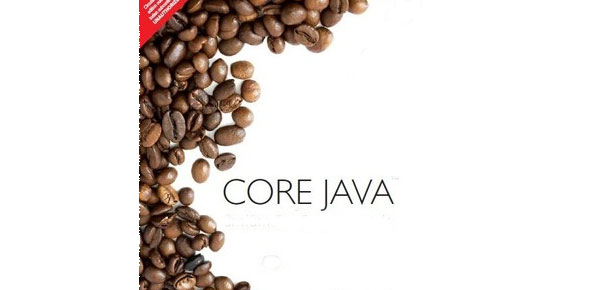# Evaluate Your Knowledge Of Core Java

5 Questions | Total Attempts: 297Settings• 1.
Which is the correct way to declare and store a character value to a variable?
• A.

Char ch="A"

• B.

Char ch='A'

• C.

Char [] ch='A'

• D.

Char []ch="A"

• 2.
How execute a java program? Programe file name is "Example.java".
• A.

Java Example

• B.

Javac Example

• C.

Java Example.java

• D.

Javac Example.java

• 3.
While key words allow us to use class variable with our declaring the object of the class?
• A.

Final

• B.

Abstract

• C.

Interface

• D.

Static

• 4.
Which of following is the correct declaration of main method?
• A.

Static public void main(String []a)

• B.

Void public static main()

• C.

Void public static main(String a[])

• D.

Public static main(String a[])

• 5.
Predict the output. abstract class cal {"\n" abstract public void sum(); abstract public void mul(); } public class calculation extens cal { int a=10,b=20,res=0; public void sum() { res=a+b; System.out.println("Result is : " +res); } public static void main(String []a) { calculation c=new calculation(); c.sum(); } }
• A.

Result is : 30

• B.

Result is : 0

• C.

Compile time error

• D.

Runtime error

Related TopicsBack to top